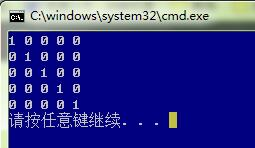• 数据表里面的字段 content 存储了一以逗号分割的字符串，最大有20个数，最大数字为40。 比如3,24,33,40类似字样的数字序列。 其实就是一保存了多项投票结果的字段啦。 现在需要统计每数字的个数，也就是每...
数据表里面的字段 content 存储了一个以逗号分割的字符串，最大有20个数，最大数字为40。
比如3,24,33,40类似字样的数字序列。
其实就是一个保存了多项投票结果的字段啦。
现在需要统计每个数字的个数，也就是每个投票项有多少人投了，并排序。
解决思路
1. 首先，从数据库的congtent字段读取数据，并把它们合并成一个字符串。
<?php
while($myrow =$connector -> fetch_array($result)) { // www.jbxue.com //$r[] = explode(",", $myrow["content"]);$str .= $myrow["content"].','; }$arr_str = substr($str, 0, -1); ?> 由于最后一个数后面有逗号，所以要对字符串进行截取。 2. 将字符串按逗号分割成数组。$r = explode(",", $arr_str); 3. 用 array_count_values() 统计一维数组的元素个数 由于array_count_values()貌似不能直接对二维数组的元素进行个数统计，所以进行了上面的两个步骤，得到一个一维数组。 array_count_values() 函数用于统计数组中所有值出现的次数。返回一个数组，其元素的键名是原数组的值，键值是该值在原数组中出现的次数。$rs = array_count_values($r); 4. 排序 asort($rs);
echo '<pre>';
print_r($rs); echo '</pre>';  展开全文• 一、两个二维数组的合并$a=Array(0 => Array("id" => 66,"class_name" => "www.iiwnet.com"),1 => Array("id" => 67,"class_name" => "linux" )); $b=Array(0 => Array("class_count"=> 8),1 => Array("class_count... 一、两个二维数组的合并 $a=Array(0 => Array("id" => 66,"class_name" => "www.iiwnet.com"),1 => Array("id" => 67,"class_name" => "linux" ));
$b=Array(0 => Array("class_count"=> 8),1 => Array("class_count" => 2));  方法一： php提供了一个方便的函数： array_merge_recursive($as_activity,$array['data']['as_activity']);//合并两个数组 方法二： 自己手动实现 $arr2 = array();

$n = 0; foreach($a as $k =>$v){
foreach($v as$k1 => $v1){$arr2[$n][$k1] = $v1; }$n++;
}
foreach($b as$k => $v){ foreach($v as $k1 =>$v1){
$arr2[$n][$k1] =$v1;
}
$n++; } 输出： array(4) {  => array(2) { ["id"] => int(66) ["class_name"] => string(14) "www.iiwnet.com" }  => array(2) { ["id"] => int(67) ["class_name"] => string(5) "linux" }  => array(1) { ["class_count"] => int(8) }  => array(1) { ["class_count"] => int(2) }} 2、php对二维数组按其中某个值，进行排序 $sort = array(
'direction' => 'SORT_DESC', //排序顺序标志 SORT_DESC 降序；SORT_ASC 升序
'field'     => 'publish_date',       //排序字段
);
$arrSort = array(); foreach($result AS $uniqid =>$row){  		//$result foreach($row AS $key=>$value){
$arrSort[$key][$uniqid] =$value;
}
}
if($sort['direction']){ array_multisort($arrSort[$sort['field']], constant($sort['direction']), $result); } 3、其他 unset($as_activity);//删除数组元素，删除后的数组，不是索引数组
$as_activity = array_values($as_activity);//重建索引数组
array_merge_recursive($as_activity,$array['data']['as_activity']);//合并两个数组array_column($array,'key')//（二维数组，二维数组的key）得到二维数组中的某一列值$res = array(); //想要的结果
foreach ($result as$k => $v) {$res[$v['time']][] =$v;
}//二维数组按相同字段合并

展开全文• 二维数组元素利用指针的三种输出方法
定义一个二维数组：

int A={{1,2},{3,4},{5,6}};

A、A、A是数组 A 第一行、第二行、第三行的起始地址，也就是说 A=&A，A=&A，A=&A。A+1 表示第二行、第二列的地址，即 &A。

利用指针，输出二维数组的元素(1)：

//利用指针，输出二维数组的元素
#include<iostream>;
using namespace std;
void main()
{
int Matrix={{1,0,0,0,0},
{0,1,0,0,0},
{0,0,1,0,0},
{0,0,0,1,0},
{0,0,0,0,1}};
int i,j,p;
for (i=0;i<5;i++)
{
for(j=0;j<5;j++)
{
p=*(Matrix[i]+j);
cout<<p<<" ";
}
cout<<endl;
}
}对于上例中的 Matrix[i] 可以看做是一维数组（即每一行的地址作为一个数组），那么Matrix[i] 还可以写成 *(Matrix+i) 的形式。因此二维数组中的元素 Matrix[i][j] 的地址还可以写成 *(Matrix+i)+j，于是Matrix[i][j] 就可以写成 *(*(Matrix+i)+j) ，同时也可写成 (*(Matrix+i))[j]。

利用指针，输出二维数组的元素(2)：

//利用指针，输出二维数组的元素(2)
#include<iostream>;
using namespace std;
void main()
{
int Matrix={{1,0,0,0,0},
{0,1,0,0,0},
{0,0,1,0,0},
{0,0,0,1,0},
{0,0,0,0,1}};
int i,j,p;
for (i=0;i<5;i++)
{
for(j=0;j<5;j++)
{
p=*(*(Matrix+i)+j);
cout<<p<<" ";
}
cout<<endl;
}
}利用指针，输出二维数组的元素(3)：

//利用指针，输出二维数组的元素(3)
#include<iostream>;
using namespace std;
void main()
{
int Matrix={{1,0,0,0,0},
{0,1,0,0,0},
{0,0,1,0,0},
{0,0,0,1,0},
{0,0,0,0,1}};
int i,j,p;
for (i=0;i<5;i++)
{
for(j=0;j<5;j++)
{
p=(*(Matrix+i))[j];
cout<<p<<" ";
}
cout<<endl;
}
}展开全文指针
• 二维数组元素表示方法 a // 数组首行的地址 a //元素a的地址。 二维数组可以看成是一维数组的数组，a 可以认为是数组名，数组名代表数组首元素的地址 a 代表 a的地址 *(a+0) //元素...
二维数组
二维数组本质上是以数组作为数组元素的数组，即“数组的数组”。
二维数组元素表示方法
 a                //  数组首行的地址

a            //元素a的地址。

二维数组可以看成是一维数组的数组，（a 、a 、a ) 。
a 可以认为是数组名，数组名代表数组首元素的地址， a 代表 a的地址

*(a+0)        //元素a的地址

因为a是二维数组名，即是一维数组（a 、a 、a )这三个元素的的首地址。
a+0就是 a的地址。
*是取指针的值。
那么*(a+0)就是取 a的值。 a 是一个数组名，他代表数组首元素a的地址。

a+1             // 数组第二行的地址

a+2       //  元素a的地址        a 是数组名，数组名是指向数组首元素的地址， a+2  数组名+2 代表数组的第三个元素的地址。

*(a+1)+2     //  元素 a的地址       a+1  就是 a 的地址 ，*(a+1)  就是 a的地址， *(a+1)+2  就是 a的地址。

*(a+2)      //元素 a  的值         a 是数组名。  a+2  是 a的地址，*(a+2) 就是 元素 a的值了。

*(*(a+1)+2）     //元素 a的值       a+1 代表 a的地址，*(a+1) 代表 a 的地址，*(a+1)+2 代表 a 的地址。



展开全文• ## 二维数组元素的地址

万次阅读 多人点赞 2017-08-17 10:18:24
维数组元素的地址大家都比较容易理解，但对于二维数组，就很容易搞混了.今天我又被这问题给弄糊涂了，翻了翻老谭的书本，对这问题有了更深的认识. 　首先给出一个二维数组a，它的定义为: 　int a = ...
• 定义了int型二维数组a后,数组元素a前的数组元素个数，叉乘加法java
• C语言总结第七章、数组一维数组一维数组的定义一维数组的引用一维数组的初始化程序举例二维数组及多维数组二维数组的定义二维数组元素的引用二维数组元素的初始化程序举例字符数组和字符串字符数组 第七章、数组 ...C语言
• 今天遇到的一问题：给出任意一个二维数组，要求输出数组元素的所有排列组合。如var myArr = [['A','B','C'],['A1','B1','C1'],['A2','B2']];myArr数组元素的所有排列组合为："AA1A2""BA1A2"&...JavaScript 排列组合
• ## 二维数组元素互换

千次阅读 2018-11-13 17:08:18
//定义数组，并给个元素赋值 int b,i,j;//定义数组和变量 printf("array a:\n"); for(i=0;i&lt;2;i++)//数组a的行数共2行，循环2次 { for(j=0;j&lt;3;j++)//3列，循...
• 输入：二维数组array、一维数组element 功能：判断array是否包含element 输出：true/false 本想着用Array.indexOf，但是数组在内存中是以引用的方式存在的，想要比较两数组是否相等，是不可以使用 a === b 来...JavaScript
•c语言
• 请编一函数： void fun(int tt[M][N],int pp[N])，tt指向一M行N列的二维数组，求出二维数组每列中最小元素，并依次放入pp所指一维数组中。二维数组中的已在主函数中赋予。 #include<conio.h> #include&...c语言
• 定义一一维数组一个二维数组如下 $fruit=array('apple','orange');$products = array( array('name'=>'apple','price'=>23.4), array('name'=>'orange','price'=>45.3), array('name'=>'biscuit','number'=>5...
• 指针与一维数组 指针移动方式访问数组元素： int a, *p; // a存放了数组的首地址 p=a; // 数组首地址赋给p p++; // 向下移动4字节 指针与数组名都是地址，因此可以混合使用访问数组元素。...
• 二维数组二维数组名   对于一位数组我们认识到其数据元素在内存中是按线性顺序依次排列的，且一维数组名的值是一指针常量。那么二维数组在内存中又是...  我们可以将一个二维数组看做是一其中元素为数组...C语言
• 例33：有一3*4的二维数组，要求用C语言实现指向元素的指针变量输出二维数组个元素的值。 解题思路：二维数组元素时整型的，它相当于整型变量，可以用int*型指针变量指向它。二维数组元素在内存中是按行顺序...c语言 指针
• 题目：给定一个二维数组判断其中是否存在某个 例如：给定数组{{1,2,8,9},{2,4,9,12},{4,7,10,13},{6,8,11,15}}，判断7是否存在于数组中我的解决算法如下：public boolean find(int[][] matrix,int rows,int ...java 算法 matrix
• 关于二维数组数组元素的存储地址，有同学问出了好问题。 　在我的课件中，出现了下面的讲解： 　a[i][j]的地址是p+(i*col+j)*d（d是数组元素所占的字节）。 　同学编程序进行验证，出问题了： 　地球停止...
• 有两种方式：1 二维数组的行列在定义的时候就是确定好的，所以编程人员是知道二维数组的大小以及行列的。 所以可以直接使用行列的值。为方便维护，可以将行列定义为宏，直接调用对应的宏名作为行列值。2 ...
• var arrayHasElement = function(array, element) { // 判断二维数组array中是否存在一维数组element for (var el of array) { if (el.length === element.length) { for (var in...
• //这种定义方法只定义了二维数组的长度，没有确定每个数组的元素个数 int[][]nums ={{30,35},{11,23,13,24},{25,21,31}};//这种定义方法直接给数组中的元素赋值 2.二维数组求和 public class Demo { pujava
• matrix = [ * 3] * 3此时matrix = [[0,2,0], [0,2,0], [0,2,0]]本意是把矩阵的第一行第列赋值为 2， 但是最终结果是每一行的第列都是 2出现这结果的原因是：list * n—&gt;n shallow copies of list ...python
• 二维数组的行数可以使用Length属性获得，但由于C#中支持不规则数组，因此二维数组中每一行中...例如，下面代码定义一不规则二维数组，并通过遍历其行数、列，输出二维数组中的内容，代码如下： 01 staticvoid MaiC# Array 遍历数组 Length
• 个二维数组中的元素和其展开后的一维数组中的元素的对应关系 二维数组a[ ][ ]是mxn维， 且int m = a.length;//rows int n = a.length;//cols 展开后的一维数组大小int[] b = new int[m*n]; 二维数组中...
• 输入一个二维数组，求二维数组中各行元素的和。 #include<iostream> using namespace std; int main() { int math,sum1,sum2; int i,j; cout<<"请输入数组元素:"<<endl; for(i=0;i<3...
• 二维数组的结构决定了其能非常方便地表示计算机中的表，以第一下标表示元素所在的行，第二下标表示元素所在的列。下面简单了解一下二维数组，包括数组的声明和初始化。 创建二维数组 在 Java 中二维数组被看作...
• 用指针变量输出二维数组元素的值。 代码： #include<stdio.h> void main(){ int a={1,2,3,4,5,6,7,8,9,10,11,12};//定义一串二维数组 int (*p)；//定义指针 p=a; int i,j; for(i=0;i<3;i++){ ...C语言
• 首先定义一个二维数组  int a; 关于 a *a **a 做出以下理解：  数组名a为数组首元素的地址 即 a第一行的地址。  *a可以理解为：将第一行看成一小数组，用*a表示，那么数组名*a为数组首元素...
• 但是如何动态分配一个二维数组呢？像这样： int **pa = new int ？测试一次 => 显然这种分配方式是不正确的： 在尝试新的方法之前，我们需要首先了解二维数组在内存中的存储结构： ......